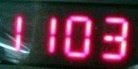## Monday, November 3, 2008

### 1103

1103 is a prime number. It is also a Sophie Germain prime. If both p and 2p + 1 are prime, then p is a Sophie Germain prime: 1103 and 2207 are both primes.

11032 = 1216609 and 30112 = 9066121.

1103 is the number of graphs with 9 vertices and 8 edges.

1103 is a number that cannot be written as a sum of three squares.

1103 is a prime whose reversal is a different prime: 3011.The Intel
1103 DRAM, introduced in 1970, was the first chip to store a significant amount of information.